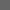HOMEDRAWING MENUFIGURE DRAWING Figure Drawing Menu
 Figure Introduction Figure Proportions Figure: Basic Poses (Easier) Figure: Foreshortening Figure: Unique Poses (Harder) Figure: Multiple Figures Figure: The Figure in Actions Figure: Advanced Figure Work Figure: Clothing for Figures